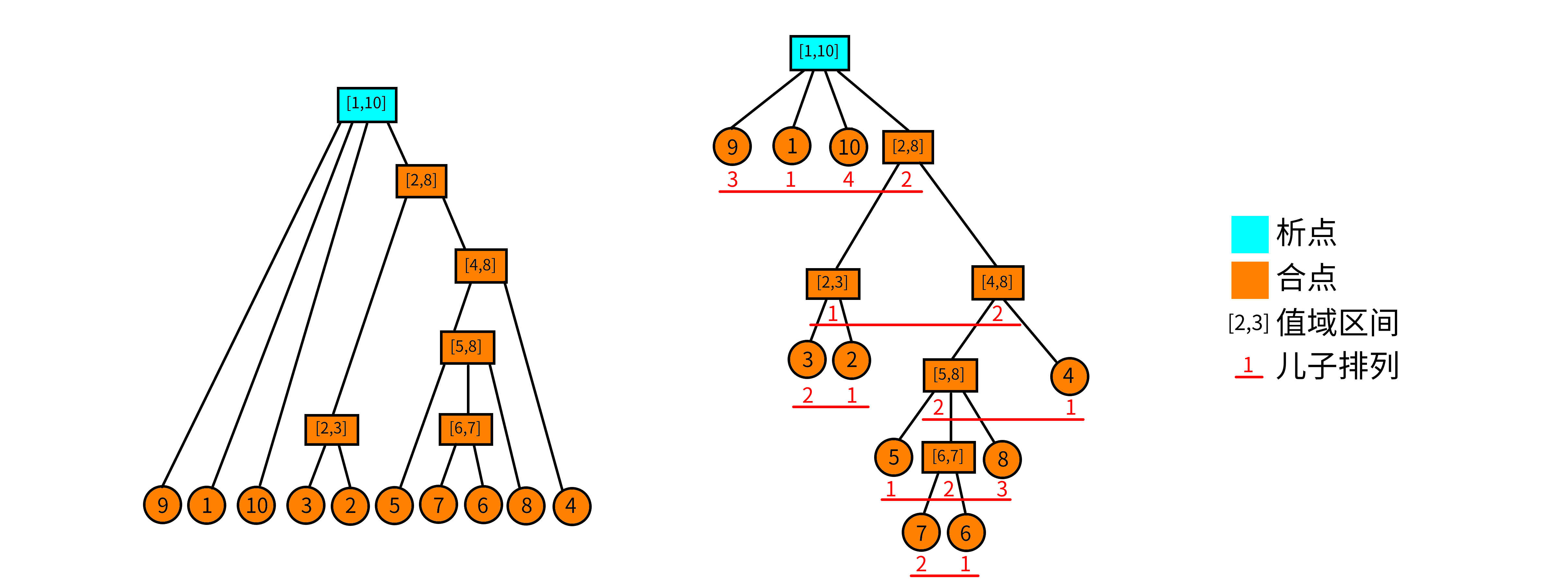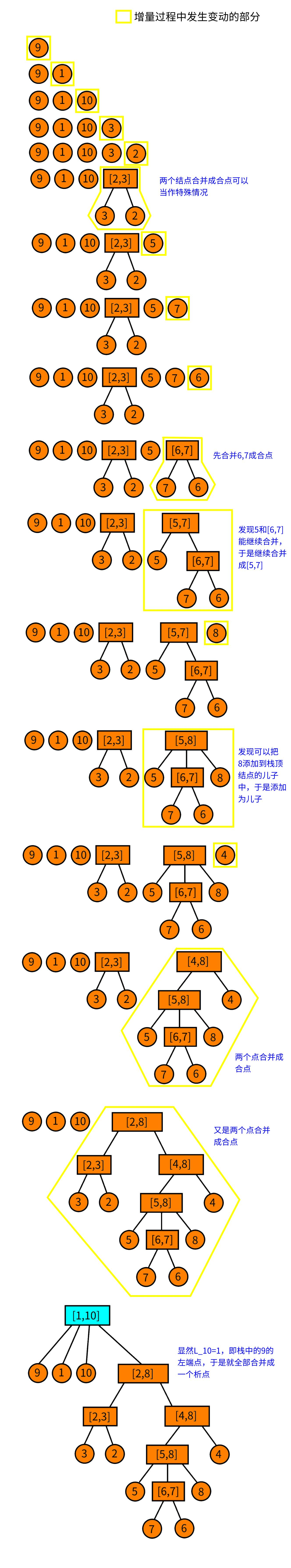# 析合树

## 连续段

### 排列与连续段

1..
2..
3..

连续段：对于排列，定义连续段表示一个区间，要求值域是连续的。说得更形式化一点，对于排列，连续段表示一个区间满足：### 连续段的运算

1..
2..
3..
4..
5..

## 析合树

### 本原段### 析点与合点

1. 值域区间：对于一个结点，用表示该结点的值域区间。
2. 儿子序列：对于析合树上的一个结点，假设它的儿子结点是一个 有序 序列，该序列是以值域区间为元素的（单个的数可以理解为的区间）。我们把这个序列称为儿子序列。记作3. 儿子排列：对于一个儿子序列，把它的元素离散化成正整数后形成的排列称为儿子排列。举个例子，对于结点，它的儿子序列为，那么把区间排序标个号，则它的儿子排列就为；类似的，结点的儿子排列为。结点的儿子排列记为4. 合点：我们认为，儿子排列为顺序或者逆序的点为合点。形式化地说，满足或者的点称为合点。叶子结点没有儿子排列，我们也认为它是合点
5. 析点：不是合点的就是析点。

### 析合树的构造

#### 增量法

1. 我们先判断它能否成为栈顶结点的儿子，如果能就变成栈顶的儿子，然后把栈顶取出，作为当前结点。重复上述过程直到栈空或者不能成为栈顶结点的儿子。
2. 如果不能成为栈顶的儿子，就看能不能把栈顶的若干个连续的结点都合并成一个结点（判断能否合并的方法在后面），把合并后的点，作为当前结点。
3. 重复上述过程直到不能进行为止。然后结束此次增量，直接把当前结点压栈。

#### 具体的策略

1. 如果不存在，那么显然当前结点无法合并；
2. 如果，那么这就是两个结点合并，合并后就是一个 合点
3. 否则在栈中一定存在一个点的左端点，那么一定可以从当前结点合并到形成一个 析点

#### 判断能否合并1. 找到最大的使得，那么显然，这一段数全部小于，于是就需要更新的最大值。由于是（非严格）单调递增的，因此可以每一段相同的做相同的更新，即区间加操作。
2. 更新同理。
3. 把每一个都减。因为区间长度加4. 查询：即查询的最小值的所在的 下标### 实现

  1 2 3 4 5 6 7 8 9 10 11 12 13 14 15 16 17 18 19 20 21 22 23 24 25 26 27 28 29 30 31 32 33 34 35 36 37 38 39 40 41 42 43 44 45 46 47 48 49 50 51 52 53 54 55 56 57 58 59 60 61 62 63 64 65 66 67 68 69 70 71 72 73 74 75 76 77 78 79 80 81 82 83 84 85 86 87 88 89 90 91 92 93 94 95 96 97 98 99 100 101 102 103 104 105 106 107 108 109 110 111 112 113 114 115 116 117 118 119 120 121 122 123 124 125 126 127 128 129 130 131 132 133 134 135 136 137 138 139 140 141 142 143 144 145 146 147 148 149 150 151 152 153 154 155 156 157 158 159 160 161 162 163 164 165 166 167 168 169 170 171 172 173 174 175 176 177 178 179 180 181 182 183 184 185 186 187 188 189 190 191 192 193 194 195 196 197 198 199 200 201 202 203 204 205 206 207 208 209 210 211 #include #define rg register using namespace std; const int N = 200010; int n, m, a[N], st1[N], st2[N], tp1, tp2, rt; int L[N], R[N], M[N], id[N], cnt, typ[N], bin, st[N], tp; // 本篇代码原题应为 CERC2017 Intrinsic Interval // a 数组即为原题中对应的排列 // st1 和 st2 分别两个单调栈，tp1、tp2 为对应的栈顶，rt 为析合树的根 // L、R 数组表示该析合树节点的左右端点，M 数组的作用在析合树构造时有提到 // id 存储的是排列中某一位置对应的节点编号，typ 用于标记析点还是合点 // st 为存储析合树节点编号的栈，tp为其栈顶 struct RMQ { // 预处理 RMQ（Max & Min） int lg[N], mn[N], mx[N]; void chkmn(int& x, int y) { if (x > y) x = y; } void chkmx(int& x, int y) { if (x < y) x = y; } void build() { for (int i = bin = 1; i < 20; ++i) bin[i] = bin[i - 1] << 1; for (int i = 2; i <= n; ++i) lg[i] = lg[i >> 1] + 1; for (int i = 1; i <= n; ++i) mn[i] = mx[i] = a[i]; for (int i = 1; i < 17; ++i) for (int j = 1; j + bin[i] - 1 <= n; ++j) mn[j][i] = min(mn[j][i - 1], mn[j + bin[i - 1]][i - 1]), mx[j][i] = max(mx[j][i - 1], mx[j + bin[i - 1]][i - 1]); } int ask_mn(int l, int r) { int t = lg[r - l + 1]; return min(mn[l][t], mn[r - bin[t] + 1][t]); } int ask_mx(int l, int r) { int t = lg[r - l + 1]; return max(mx[l][t], mx[r - bin[t] + 1][t]); } } D; // 维护 L_i struct SEG { // 线段树 #define ls (k << 1) #define rs (k << 1 | 1) int mn[N << 1], ly[N << 1]; // 区间加；区间最小值 void pushup(int k) { mn[k] = min(mn[ls], mn[rs]); } void mfy(int k, int v) { mn[k] += v, ly[k] += v; } void pushdown(int k) { if (ly[k]) mfy(ls, ly[k]), mfy(rs, ly[k]), ly[k] = 0; } void update(int k, int l, int r, int x, int y, int v) { if (l == x && r == y) { mfy(k, v); return; } pushdown(k); int mid = (l + r) >> 1; if (y <= mid) update(ls, l, mid, x, y, v); else if (x > mid) update(rs, mid + 1, r, x, y, v); else update(ls, l, mid, x, mid, v), update(rs, mid + 1, r, mid + 1, y, v); pushup(k); } int query(int k, int l, int r) { // 询问 0 的位置 if (l == r) return l; pushdown(k); int mid = (l + r) >> 1; if (!mn[ls]) return query(ls, l, mid); else return query(rs, mid + 1, r); // 如果不存在 0 的位置就会自动返回当前你查询的位置 } } T; int o = 1, hd[N], dep[N], fa[N]; struct Edge { int v, nt; } E[N << 1]; void add(int u, int v) { // 树结构加边 E[o] = (Edge){v, hd[u]}; hd[u] = o++; } void dfs(int u) { for (int i = 1; bin[i] <= dep[u]; ++i) fa[u][i] = fa[fa[u][i - 1]][i - 1]; for (int i = hd[u]; i; i = E[i].nt) { int v = E[i].v; dep[v] = dep[u] + 1; fa[v] = u; dfs(v); } } int go(int u, int d) { for (int i = 0; i < 18 && d; ++i) if (bin[i] & d) d ^= bin[i], u = fa[u][i]; return u; } int lca(int u, int v) { if (dep[u] < dep[v]) swap(u, v); u = go(u, dep[u] - dep[v]); if (u == v) return u; for (int i = 17; ~i; --i) if (fa[u][i] != fa[v][i]) u = fa[u][i], v = fa[v][i]; return fa[u]; } // 判断当前区间是否为连续段 bool judge(int l, int r) { return D.ask_mx(l, r) - D.ask_mn(l, r) == r - l; } // 建树 void build() { for (int i = 1; i <= n; ++i) { // 单调栈 // 在区间 [st1[tp1-1]+1,st1[tp1]] 的最小值就是 a[st1[tp1]] // 现在把它出栈，意味着要把多减掉的 Min 加回来。 // 线段树的叶结点位置 j 维护的是从 j 到当前的 i 的 // Max{j,i}-Min{j,i}-(i-j) // 区间加只是一个 Tag。 // 维护单调栈的目的是辅助线段树从 i-1 更新到 i。 // 更新到 i 后，只需要查询全局最小值即可知道是否有解 while (tp1 && a[i] <= a[st1[tp1]]) // 单调递增的栈，维护 Min T.update(1, 1, n, st1[tp1 - 1] + 1, st1[tp1], a[st1[tp1]]), tp1--; while (tp2 && a[i] >= a[st2[tp2]]) T.update(1, 1, n, st2[tp2 - 1] + 1, st2[tp2], -a[st2[tp2]]), tp2--; T.update(1, 1, n, st1[tp1] + 1, i, -a[i]); st1[++tp1] = i; T.update(1, 1, n, st2[tp2] + 1, i, a[i]); st2[++tp2] = i; id[i] = ++cnt; L[cnt] = R[cnt] = i; // 这里的 L,R 是指节点所对应区间的左右端点 int le = T.query(1, 1, n), now = cnt; while (tp && L[st[tp]] >= le) { if (typ[st[tp]] && judge(M[st[tp]], i)) { // 判断是否能成为儿子，如果能就做 R[st[tp]] = i, M[st[tp]] = L[now], add(st[tp], now), now = st[tp--]; } else if (judge(L[st[tp]], i)) { typ[++cnt] = 1; // 合点一定是被这样建出来的 L[cnt] = L[st[tp]], R[cnt] = i, M[cnt] = L[now]; // 这里M数组是记录节点最右面的儿子的左端点，用于上方能否成为儿子的判断 add(cnt, st[tp--]), add(cnt, now); now = cnt; } else { add(++cnt, now); // 新建一个结点，把 now 添加为儿子 // 如果从当前结点开始不能构成连续段，就合并。 // 直到找到一个结点能构成连续段。而且我们一定能找到这样 // 一个结点。 do add(cnt, st[tp--]); while (tp && !judge(L[st[tp]], i)); L[cnt] = L[st[tp]], R[cnt] = i, add(cnt, st[tp--]); now = cnt; } } st[++tp] = now; // 增量结束，把当前点压栈 T.update(1, 1, n, 1, i, -1); // 因为区间右端点向后移动一格，因此整体 -1 } rt = st; // 栈中最后剩下的点是根结点 } void query(int l, int r) { int x = id[l], y = id[r]; int z = lca(x, y); if (typ[z] & 1) l = L[go(x, dep[x] - dep[z] - 1)], r = R[go(y, dep[y] - dep[z] - 1)]; // 合点这里特判的原因是因为这个合点不一定是最小的包含l，r的连续段. // 因为合点所代表的区间的子区间也都是连续段，而我们只需要其中的一段就够了。 else l = L[z], r = R[z]; printf("%d %d\n", l, r); } // 分 lca 为析或和，这里把叶子看成析的 int main() { scanf("%d", &n); for (int i = 1; i <= n; ++i) scanf("%d", &a[i]); D.build(); build(); dfs(rt); scanf("%d", &m); for (int i = 1; i <= m; ++i) { int x, y; scanf("%d%d", &x, &y); query(x, y); } return 0; } // 20190612 // 析合树 

## 参考文献与链接

1. 刘承奥。简单的连续段数据结构。WC2019 营员交流。